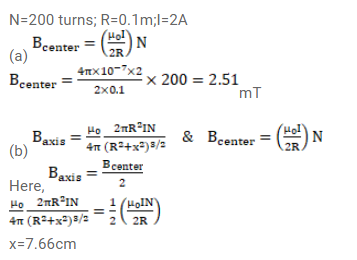# A circular coil of 200 turns has a radius of

Question:

A circular coil of 200 turns has a radius of $10 \mathrm{~cm}$ and carries a current of $2.0 \mathrm{~A}$.

(a) Find the magnitude of the magnetic field $\overrightarrow{\mathrm{B}}$ at the center of the coil.

(b) At what distance from the center along the axis of the coil will the field B drop to half its value at the center? ( $\sqrt{4}=1.5874 \ldots .)$

Solution: